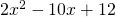Chapter 7: Factoring

# 7.7 Choosing the Correct Factoring Strategy

With so many different tools used to factor, it is prudent to have a section to determine the best strategy to factor.

## Factoring Hints

1. Look for any factor to simplify the polynomial before you start!
2. If you have two terms, look for a sum or difference of squares or cubes.
•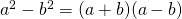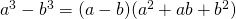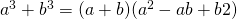3. If you have three terms, see if the master product method works.
4. If you have four terms, see if factoring by grouping works.

If you find that you have trouble factoring the questions in this section, then you might need to redo from the start of Chapter 7. This is not uncommon.

# Questions

Factor each completely.

1.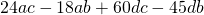2.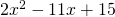3.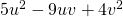4.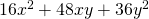5.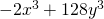6.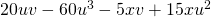7.8.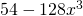9.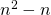10.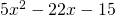11.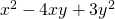12.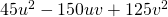13.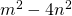14.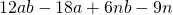15.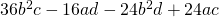16.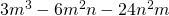17.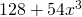18.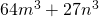19.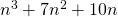20.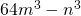21.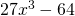22.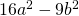23.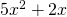24.